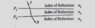# Physics question: index of refraction

• YMMMA
Same direction" means same angle from the normal line. And "same direction in space" means same angle from the normal line, irrespective of normals or planes. So if the speeds are equal, the rays will be parallel.

## Homework Statement

[/B]
In order for the refracted ray R to have the same direction in space as the incident ray I, shown above, which of the following conditions by itself would be sufficient? (A) n1=n2 (B) n1=n3 (C) n2=n3 (D) Plane surface P, is parallel to plane surface P2- (E) None of the conditions above would be sufficient by itself.

## Homework Equations

Index of refraction = speed of light / speed v

## The Attempt at a Solution

I thought same direction means same angle from the normal line ,and for that to happen, their speeds must be equal. I am not sure if that’s correct ,but I answered it E, anyway. Am I right?

#### Attachments

•4E07CBFB-D46B-46C2-B698-6D36F5238D3D.jpeg
13.4 KB · Views: 412
Last edited:
YMMMA said:
I thought same direction means same angle from the normal line,...
That's correct.

YMMMA said:
and for that to happen, their speeds must be equal.
"Speeds equal" would be n1 = n3. But it's not sufficient. As a simple counterexample, consider a prism in air. The speed of the ray going out is the same as the speed coming in, but the angle is NOT the same.

Why not? Because the normals of the two surfaces are not pointing in the same direction. If the refracted ray is making an angle of 30 degrees with the normal at the n1/n2 surface, and the n2/n3 surface is in a different direction, then it's not making an angle of 30 degrees when it hits that surface. Maybe it's 25 degrees. Maybe it's 50 degrees. And as a result, even if n1 = n3, the outgoing angle is going to be different from the original angle.

Again, think of a prism.

So you also need the condition that the two planes are parallel, choice D. But that's not sufficient on its own either, if the indexes n1 and n3 are different.

YMMMA said:
I am not sure if that’s correct ,but I answered it E, anyway. Am I right?
Yes, because neither (B) nor (D) suffices on its own, but if both are true, the outgoing ray will be parallel to the incoming ray.

Right answer, but I'm not sure if your reasoning was 100% correct.

That’s convincing enough. Thanks for your clarification.

YMMMA said:
...

## Homework Equations

Index of refraction = speed of light / speed v I don't see the relevance of this

...
which conditions by itself would be sufficient?
(A) n1=n2 ⇒ no deviation at first surface, but could have any deviation at second
(B) n1=n3 ⇒ deviations equal, if surfaces are parallel: not otherwise
(C) n2=n3 ⇒ no deviation at second surface, but could have any deviation at first
(D) Plane surface P, is parallel to plane surface P2- ⇒ no overall deviation only if n1=n3
(E) None of the conditions above would be sufficient by itself.

## The Attempt at a Solution

I thought same direction means same angle from the normal line , I think "same direction in space" means entry ray is parallel to output ray, irrespective of normals or planes
and for that to happen, their speeds must be equal. speeds will be equal only if n1=n3, but the rays may or may not be parallel

Understood. Thanks a million.